##### Geometry For Dummies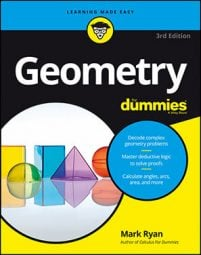What follows are over three dozen of the most important geometry formulas, theorems, properties, and so on that you use for calculations. If you get stumped while working on a geometry problem and can’t come up with a formula, this is the place to look.

## Circle stuff

• Circumference: C = 2πr or πd, where r is the radius of the circle and d is its diameter
• Area: AreaCircle = πr2
• Arc length: The length of an arc (part of the circumference) is equal to the circumference of the circle (2πr) times the fraction of the circle represented by the arc.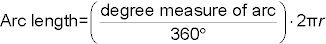• Sector area: The area of a sector (a pizza-slice shape cut out of a circle) is equal to the area of the circle (πr2) times the fraction of the circle represented by the sector.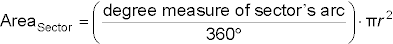• Measure of an angle . . .
• Chord-Chord Power Theorem: When two chords of a circle intersect, the product of the parts of one chord is equal to the product of the parts of the other chord.
• Tangent-Secant Power Theorem: When a tangent and a secant of a circle meet at an external point, the measure of the tangent squared is equal to the product of the secant’s external part and its total length.
• Secant-Secant Power Theorem: When two secants of a circle meet at an external point, the product of one secant’s external part and its total length is equal to the product of the other secant’s external part and its total length.

## 3-D geometry stuff

• Flat-top objects (prisms and cylinders):
• Volume: VolFlat-top = areabase × height
• Surface area: SAFlat-top = 2 × areabase + arealateral rectangles For a cylinder, the single lateral rectangle that wraps around the cylinder has a length equal to the circumference of the cylinder’s base; its width is the cylinder’s height.
• Pointy-top objects (pyramids and cones):
• Volume: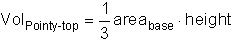• Surface area: SAPointy-top = areabase + arealateral triangles For a pyramid, the base of each lateral triangle is a side of the pyramid’s base, and the height of the triangle is the slant height of the pyramid. For a cone, one “triangle” wraps around the cone; its base is equal to the circumference of the cone’s base, and its height is equal to the slant height of the cone.
• Sphere:

## Coordinate geometry stuff

• Slope formula: Given two points (x1, y1) and (x2, y2), the slope of the line that goes through the points is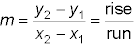It doesn’t matter which point is designated as (x1, y1) and which is designated as (x2, y2).
• The slopes of parallel lines are equal.
• The slopes of perpendicular lines are opposite reciprocals of each other.
• Midpoint formula: Given a segment with endpoints (x1, y1) and (x2, y2), the coordinates of its midpoint are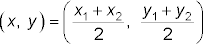It doesn’t matter which point is (x1, y1) and which is(x2, y2).
• Distance formula: Given two points (x1, y1) and (x2, y2), the distance between the points is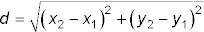It doesn’t matter which point is (x1, y1) and which is (x2, y2).
• Equations of a line:
• Slope-intercept form: y = mx + b, where m is the slope and b is the y-intercept
• Point-slope form: yy1 = m(xx1), where m is the slope and (x1, y1) is a point on the line
• Horizontal line: y = b, where b is the y-intercept
• Vertical line: x = a, where a is the x-intercept
• Equation of a circle: (xh)2 + (yk)2 = r2, where (h, k) is the center of the circle and r is its radius